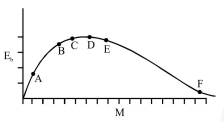# The above is a plot of binding energy per nucleon

Question:The above is a plot of binding energy per nucleon $\mathrm{E}_{\mathrm{b}}$, against the nuclear mass $\mathrm{M} ; \mathrm{A}, \mathrm{B}, \mathrm{C}, \mathrm{D}, \mathrm{E}, \mathrm{F}$ correspond to different nuclei. Consider four reactions:

(i) $\mathrm{A}+\mathrm{B} \rightarrow \mathrm{C}+\varepsilon$

(ii) $\mathrm{C} \rightarrow \mathrm{A}+\mathrm{B}+\varepsilon$

(iii) $\mathrm{D}+\mathrm{E} \rightarrow \mathrm{F}+\varepsilon$

(iv) $\mathrm{F} \rightarrow \mathrm{D}+\mathrm{E}+\varepsilon$

where $\varepsilon$ is the energy released ? In which reactions is $\varepsilon$ positive ?

1. (ii) and (iv)

2. (ii) and (iii)

3. (i) and (iv)

4. (i) and (iii)

Correct Option: , 3

Solution:

If B.E/A increases then Energy released in the reaction.